## 1.1 Review of Functions

### Learning Objectives

• Use functional notation to evaluate a function.
• Determine the domain and range of a function.
• Draw the graph of a function.
• Find the zeros of a function.
• Recognize a function from a table of values.
• Make new functions from two or more given functions.
• Describe the symmetry properties of a function.

In this section, we provide a formal definition of a function and examine several ways in which functions are represented—namely, through tables, formulas, and graphs. We study formal notation and terms related to functions. We also define composition of functions and symmetry properties. Most of this material will be a review for you, but it serves as a handy reference to remind you of some of the algebraic techniques useful for working with functions.

# Functions

Given two sets $A$ and $B$, a set with elements that are ordered pairs $(x,y)$, where $x$ is an element of $A$ and $y$ is an element of $B$, is a relation from $A$ to $B$. A relation from $A$ to $B$ defines a relationship between those two sets. A function is a special type of relation in which each element of the first set is related to exactly one element of the second set. The element of the first set is called the input; the element of the second set is called the output. Functions are used all the time in mathematics to describe relationships between two sets. For any function, when we know the input, the output is determined, so we say that the output is a function of the input. For example, the area of a square is determined by its side length, so we say that the area (the output) is a function of its side length (the input). The velocity of a ball thrown in the air can be described as a function of the amount of time the ball is in the air. The cost of mailing a package is a function of the weight of the package. Since functions have so many uses, it is important to have precise definitions and terminology to study them.

### Definition

A function $f$ consists of a set of inputs, a set of outputs, and a rule for assigning each input to exactly one output. The set of inputs is called the domain of the function. The set of outputs is called the range of the function.

For example, consider the function $f$, where the domain is the set of all real numbers and the rule is to square the input. Then, the input $x=3$ is assigned to the output $3^2=9$. Since every nonnegative real number has a real-value square root, every nonnegative number is an element of the range of this function. Since there is no real number with a square that is negative, the negative real numbers are not elements of the range. We conclude that the range is the set of nonnegative real numbers.

For a general function $f$ with domain $D$, we often use $x$ to denote the input and $y$ to denote the output associated with $x$. When doing so, we refer to $x$ as the independent variable and $y$ as the dependent variable, because it depends on $x$. Using function notation, we write $y=f(x)$, and we read this equation as “$y$ equals $f$ of $x$.” For the squaring function described earlier, we write $f(x)=x^2$.

The concept of a function can be visualized using (Figure), (Figure), and (Figure).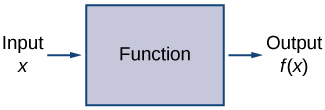Figure 1. A function can be visualized as an input/output device.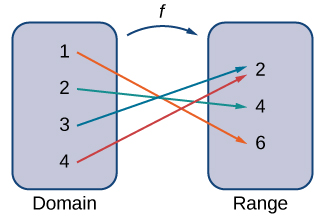Figure 2. A function maps every element in the domain to exactly one element in the range. Although each input can be sent to only one output, two different inputs can be sent to the same output.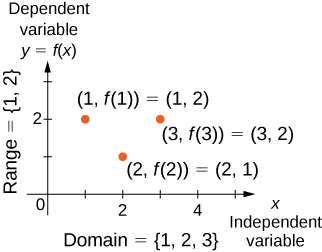Figure 3. In this case, a graph of a function $f$ has a domain of $\{1,2,3\}$ and a range of $\{1,2\}$. The independent variable is $x$ and the dependent variable is $y$.

Visit this applet link to see more about graphs of functions.

We can also visualize a function by plotting points $(x,y)$ in the coordinate plane where $y=f(x)$. The graph of a function is the set of all these points. For example, consider the function $f$, where the domain is the set $D=\{1,2,3\}$ and the rule is $f(x)=3-x$. In (Figure), we plot a graph of this function.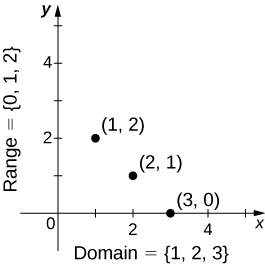Figure 4. Here we see a graph of the function $f$ with domain $\{1,2,3\}$ and rule $f(x)=3-x$. The graph consists of the points $(x,f(x))$ for all $x$ in the domain.

Every function has a domain. However, sometimes a function is described by an equation, as in $f(x)=x^2$, with no specific domain given. In this case, the domain is taken to be the set of all real numbers $x$ for which $f(x)$ is a real number. For example, since any real number can be squared, if no other domain is specified, we consider the domain of $f(x)=x^2$ to be the set of all real numbers. On the other hand, the square root function $f(x)=\sqrt{x}$ only gives a real output if $x$ is nonnegative. Therefore, the domain of the function $f(x)=\sqrt{x}$ is the set of nonnegative real numbers, sometimes called the natural domain.

For the functions $f(x)=x^2$ and $f(x)=\sqrt{x}$, the domains are sets with an infinite number of elements. Clearly we cannot list all these elements. When describing a set with an infinite number of elements, it is often helpful to use set-builder or interval notation. When using set-builder notation to describe a subset of all real numbers, denoted $ℝ$, we write

$\{x|x \, \text{has some property}\}$.

We read this as the set of real numbers $x$ such that $x$ has some property. For example, if we were interested in the set of real numbers that are greater than one but less than five, we could denote this set using set-builder notation by writing

$\{x|1<x<5\}$.

A set such as this, which contains all numbers greater than $a$ and less than $b$, can also be denoted using the interval notation $(a,b)$. Therefore,

$(1,5)=\{x|1<x<5\}$.

The numbers 1 and 5 are called the endpoints of this set. If we want to consider the set that includes the endpoints, we would denote this set by writing

$[1,5]=\{x|1\le x\le 5\}$.

We can use similar notation if we want to include one of the endpoints, but not the other. To denote the set of nonnegative real numbers, we would use the set-builder notation

$\{x|0\le x\}$.

The smallest number in this set is zero, but this set does not have a largest number. Using interval notation, we would use the symbol $\infty$, which refers to positive infinity, and we would write the set as

$[0,\infty)=\{x|0\le x\}$.

It is important to note that $\infty$ is not a real number. It is used symbolically here to indicate that this set includes all real numbers greater than or equal to zero. Similarly, if we wanted to describe the set of all nonpositive numbers, we could write

$(−\infty ,0]=\{x|x\le 0\}$.

Here, the notation $−\infty$ refers to negative infinity, and it indicates that we are including all numbers less than or equal to zero, no matter how small. The set

$(−\infty ,\infty)=\{x|x \, \text{is any real number}\}$

refers to the set of all real numbers.

Some functions are defined using different equations for different parts of their domain. These types of functions are known as piecewise-defined functions. For example, suppose we want to define a function $f$ with a domain that is the set of all real numbers such that $f(x)=3x+1$ for $x\ge 2$ and $f(x)=x^2$ for $x<2$. We denote this function by writing

$f(x)=\begin{cases} 3x+1, & x \ge 2 \\ x^2, & x < 2 \end{cases}$.

When evaluating this function for an input $x$, the equation to use depends on whether $x\ge 2$ or $x<2$. For example, since $5>2$, we use the fact that $f(x)=3x+1$ for $x\ge 2$ and see that $f(5)=3(5)+1=16$. On the other hand, for $x=-1$, we use the fact that $f(x)=x^2$ for $x<2$ and see that $f(-1)=1$.

### Evaluating Functions

For the function $f(x)=3x^2+2x-1$, evaluate

1. $f(-2)$
2. $f(\sqrt{2})$
3. $f(a+h)$

For $f(x)=x^2-3x+5$, evaluate $f(1)$ and $f(a+h)$.

#### Hint

Substitute 1 and $a+h$ for $x$ in the formula for $f(x)$.

### Finding Domain and Range

For each of the following functions, determine the i. domain and ii. range.

1. $f(x)=(x-4)^2+5$
2. $f(x)=\sqrt{3x+2}-1$
3. $f(x)=\frac{3}{x-2}$

Find the domain and range for $f(x)=\sqrt{4-2x}+5$.

#### Hint

Use $4-2x\ge 0$.

# Representing Functions

Typically, a function is represented using one or more of the following tools:

• A table
• A graph
• A formula

We can identify a function in each form, but we can also use them together. For instance, we can plot on a graph the values from a table or create a table from a formula.

## Tables

Functions described using a table of values arise frequently in real-world applications. Consider the following simple example. We can describe temperature on a given day as a function of time of day. Suppose we record the temperature every hour for a 24-hour period starting at midnight. We let our input variable $x$ be the time after midnight, measured in hours, and the output variable $y$ be the temperature $x$ hours after midnight, measured in degrees Fahrenheit. We record our data in (Figure).

Temperature as a Function of Time of Day
Hours after Midnight Temperature $(\text{°}F)$ Hours after Midnight Temperature $(\text{°}F)$
0 58 12 84
1 54 13 85
2 53 14 85
3 52 15 83
4 52 16 82
5 55 17 80
6 60 18 77
7 64 19 74
8 72 20 69
9 75 21 65
10 78 22 60
11 80 23 58

We can see from the table that temperature is a function of time, and the temperature decreases, then increases, and then decreases again. However, we cannot get a clear picture of the behavior of the function without graphing it.

## Graphs

Given a function $f$ described by a table, we can provide a visual picture of the function in the form of a graph. Graphing the temperatures listed in (Figure) can give us a better idea of their fluctuation throughout the day. (Figure) shows the plot of the temperature function.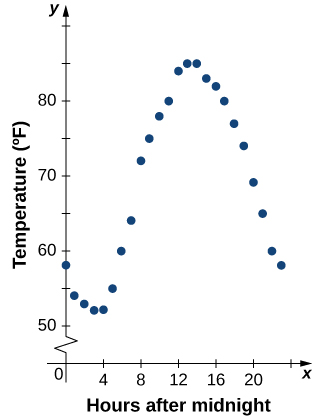Figure 5. The graph of the data from (Table) shows temperature as a function of time.

From the points plotted on the graph in (Figure), we can visualize the general shape of the graph. It is often useful to connect the dots in the graph, which represent the data from the table. In this example, although we cannot make any definitive conclusion regarding what the temperature was at any time for which the temperature was not recorded, given the number of data points collected and the pattern in these points, it is reasonable to suspect that the temperatures at other times followed a similar pattern, as we can see in (Figure).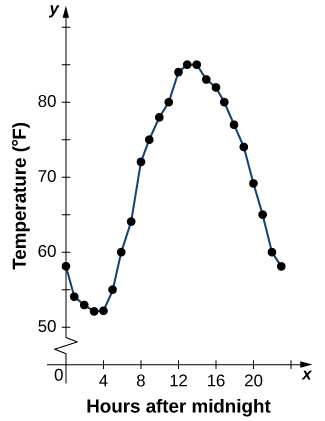Figure 6. Connecting the dots in (Figure) shows the general pattern of the data.

## Algebraic Formulas

Sometimes we are not given the values of a function in table form, rather we are given the values in an explicit formula. Formulas arise in many applications. For example, the area of a circle of radius $r$ is given by the formula $A(r)=\pi r^2$. When an object is thrown upward from the ground with an initial velocity $v_0$ ft/s, its height above the ground from the time it is thrown until it hits the ground is given by the formula $s(t)=-16t^2+v_0t$. When $P$ dollars are invested in an account at an annual interest rate $r$ compounded continuously, the amount of money after $t$ years is given by the formula $A(t)=Pe^{rt}$. Algebraic formulas are important tools to calculate function values. Often we also represent these functions visually in graph form.

Given an algebraic formula for a function $f$, the graph of $f$ is the set of points $(x,f(x))$, where $x$ is in the domain of $f$ and $f(x)$ is in the range. To graph a function given by a formula, it is helpful to begin by using the formula to create a table of inputs and outputs. If the domain of $f$ consists of an infinite number of values, we cannot list all of them, but because listing some of the inputs and outputs can be very useful, it is often a good way to begin.

When creating a table of inputs and outputs, we typically check to determine whether zero is an output. Those values of $x$ where $f(x)=0$ are called the zeros of a function. For example, the zeros of $f(x)=x^2-4$ are $x=±2$. The zeros determine where the graph of $f$ intersects the $x$-axis, which gives us more information about the shape of the graph of the function. The graph of a function may never intersect the $x$-axis, or it may intersect multiple (or even infinitely many) times.

Another point of interest is the $y$-intercept, if it exists. The $y$-intercept is given by $(0,f(0))$.

Since a function has exactly one output for each input, the graph of a function can have, at most, one $y$-intercept. If $x=0$ is in the domain of a function $f$, then $f$ has exactly one $y$-intercept. If $x=0$ is not in the domain of $f$, then $f$ has no $y$-intercept. Similarly, for any real number $c$, if $c$ is in the domain of $f$, there is exactly one output $f(c)$, and the line $x=c$ intersects the graph of $f$ exactly once. On the other hand, if $c$ is not in the domain of $f$, $f(c)$ is not defined and the line $x=c$ does not intersect the graph of $f$. This property is summarized in the vertical line test.

### Rule: Vertical Line Test

Given a function $f$, every vertical line that may be drawn intersects the graph of $f$ no more than once. If any vertical line intersects a set of points more than once, the set of points does not represent a function.

We can use this test to determine whether a set of plotted points represents the graph of a function ((Figure)).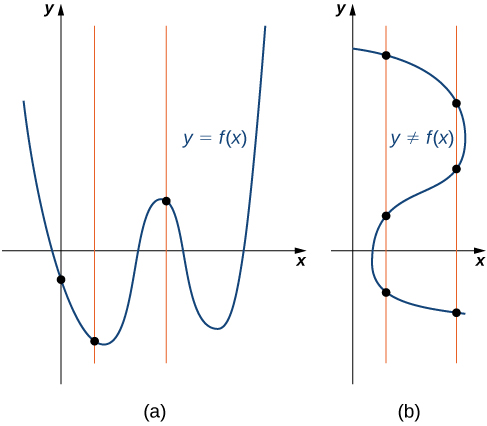Figure 7. (a) The set of plotted points represents the graph of a function because every vertical line intersects the set of points, at most, once. (b) The set of plotted points does not represent the graph of a function because some vertical lines intersect the set of points more than once.

### Finding Zeros and $y$-Intercepts of a Function

Consider the function $f(x)=-4x+2$.

1. Find all zeros of $f$.
2. Find the $y$-intercept (if any).
3. Sketch a graph of $f$.

### Using Zeros and $y$-Intercepts to Sketch a Graph

Consider the function $f(x)=\sqrt{x+3}+1$.

1. Find all zeros of $f$.
2. Find the $y$-intercept (if any).
3. Sketch a graph of $f$.

Find the zeros of $f(x)=x^3-5x^2+6x$.

#### Hint

Factor the polynomial.

### Finding the Height of a Free-Falling Object

If a ball is dropped from a height of 100 ft, its height $s$ at time $t$ is given by the function $s(t)=-16t^2+100$, where $s$ is measured in feet and $t$ is measured in seconds. The domain is restricted to the interval $[0,c]$, where $t=0$ is the time when the ball is dropped and $t=c$ is the time when the ball hits the ground.

1. Create a table showing the height $s(t)$ when $t=0,0.5,1,1.5,2,$ and $2.5$. Using the data from the table, determine the domain for this function. That is, find the time $c$ when the ball hits the ground.
2. Sketch a graph of $s$.

Note that for this function and the function $f(x)=-4x+2$ graphed in (Figure), the values of $f(x)$ are getting smaller as $x$ is getting larger. A function with this property is said to be decreasing. On the other hand, for the function $f(x)=\sqrt{x+3}+1$ graphed in (Figure), the values of $f(x)$ are getting larger as the values of $x$ are getting larger. A function with this property is said to be increasing. It is important to note, however, that a function can be increasing on some interval or intervals and decreasing over a different interval or intervals. For example, using our temperature function in (Figure), we can see that the function is decreasing on the interval $(0,4)$, increasing on the interval $(4,14)$, and then decreasing on the interval $(14,23)$. We make the idea of a function increasing or decreasing over a particular interval more precise in the next definition.

### Definition

We say that a function $f$ is increasing on the interval $I$ if for all $x_1, x_2\in I$,

$f(x_1)\le f(x_2) \, \text{when} \, x_1<x_2$.

We say $f$ is strictly increasing on the interval $I$ if for all $x_1,x_2\in I$,

$f(x_1)<f(x_2) \, \text{when} \, x_1<x_2$.

We say that a function $f$ is decreasing on the interval $I$ if for all $x_1, x_2\in I$,

$f(x_1)\ge f(x_2) \, \text{if} \, x_1<x_2$.

We say that a function $f$ is strictly decreasing on the interval $I$ if for all $x_1, x_2 \in I$,

$f(x_1)>f(x_2) \, \text{if} \, x_1<x_2$.

For example, the function $f(x)=3x$ is increasing on the interval $(−\infty ,\infty)$ because $3x_1<3x_2$ whenever $x_1<x_2$. On the other hand, the function $f(x)=−x^3$ is decreasing on the interval $(−\infty ,\infty)$ because $−(x_1)^3>-(x_2)^3$ whenever $x_1<x_2$ ((Figure)).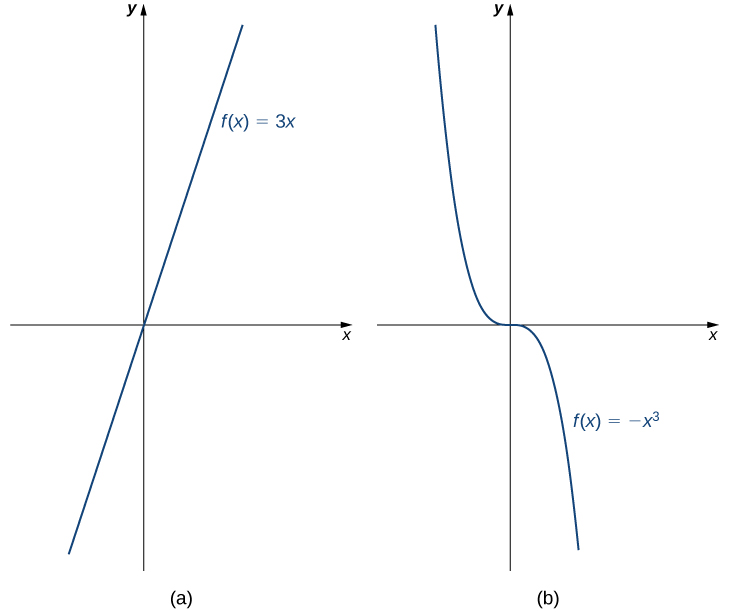Figure 10. (a) The function $f(x)=3x$ is increasing on the interval $(−\infty ,\infty)$. (b) The function $f(x)=−x^3$ is decreasing on the interval $(−\infty ,\infty)$.

# Combining Functions

Now that we have reviewed the basic characteristics of functions, we can see what happens to these properties when we combine functions in different ways, using basic mathematical operations to create new functions. For example, if the cost for a company to manufacture $x$ items is described by the function $C(x)$ and the revenue created by the sale of $x$ items is described by the function $R(x)$, then the profit on the manufacture and sale of $x$ items is defined as $P(x)=R(x)-C(x)$. Using the difference between two functions, we created a new function.

Alternatively, we can create a new function by composing two functions. For example, given the functions $f(x)=x^2$ and $g(x)=3x+1$, the composite function $f\circ g$ is defined such that

$(f\circ g)(x)=f(g(x))=(g(x))^2=(3x+1)^2$.

The composite function $g\circ f$ is defined such that

$(g\circ f)(x)=g(f(x))=3f(x)+1=3x^2+1$.

Note that these two new functions are different from each other.

## Combining Functions with Mathematical Operators

To combine functions using mathematical operators, we simply write the functions with the operator and simplify. Given two functions $f$ and $g,$ we can define four new functions:

$\begin{array}{cccc}(f+g)(x)=f(x)+g(x)\hfill & & & \text{Sum}\hfill \\ (f-g)(x)=f(x)-g(x)\hfill & & & \text{Difference}\hfill \\ (f·g)(x)=f(x)g(x)\hfill & & & \text{Product}\hfill \\ \Big(\frac{f}{g}\Big)(x)=\frac{f(x)}{g(x)} \, \text{for} \, g(x)\ne 0\hfill & & & \text{Quotient}\hfill \end{array}$

### Combining Functions Using Mathematical Operations

Given the functions $f(x)=2x-3$ and $g(x)=x^2-1$, find each of the following functions and state its domain.

1. $(f+g)(x)$
2. $(f-g)(x)$
3. $(f·g)(x)$
4. $\Big(\frac{f}{g}\Big)(x)$

For $f(x)=x^2+3$ and $g(x)=2x-5$, find $(f/g)(x)$ and state its domain.

#### Hint

The new function $(f/g)(x)$ is a quotient of two functions. For what values of $x$ is the denominator zero?

## Function Composition

When we compose functions, we take a function of a function. For example, suppose the temperature $T$ on a given day is described as a function of time $t$ (measured in hours after midnight) as in (Figure). Suppose the cost $C$, to heat or cool a building for 1 hour, can be described as a function of the temperature $T$. Combining these two functions, we can describe the cost of heating or cooling a building as a function of time by evaluating $C(T(t))$. We have defined a new function, denoted $C\circ T$, which is defined such that $(C\circ T)(t)=C(T(t))$ for all $t$ in the domain of $T$. This new function is called a composite function. We note that since cost is a function of temperature and temperature is a function of time, it makes sense to define this new function $(C\circ T)(t)$. It does not make sense to consider $(T\circ C)(t)$, because temperature is not a function of cost.

### Definition

Consider the function $f$ with domain $A$ and range $B$, and the function $g$ with domain $D$ and range $E$. If $B$ is a subset of $D$, then the composite function $(g\circ f)(x)$ is the function with domain $A$ such that

$(g\circ f)(x)=g(f(x))$.

A composite function $g\circ f$ can be viewed in two steps. First, the function $f$ maps each input $x$ in the domain of $f$ to its output $f(x)$ in the range of $f$. Second, since the range of $f$ is a subset of the domain of $g$, the output $f(x)$ is an element in the domain of $g$, and therefore it is mapped to an output $g(f(x))$ in the range of $g$. In (Figure), we see a visual image of a composite function.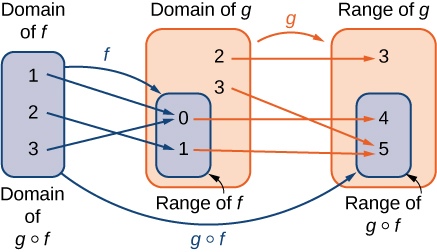Figure 11. For the composite function $g\circ f$, we have $(g\circ f)(1)=4, \, (g\circ f)(2)=5,$ and $(g\circ f)(3)=4$.

### Compositions of Functions Defined by Formulas

Consider the functions $f(x)=x^2+1$ and $g(x)=1/x$.

1. Find $(g\circ f)(x)$ and state its domain and range.
2. Evaluate $(g\circ f)(4)$ and $(g\circ f)(-1/2)$.
3. Find $(f\circ g)(x)$ and state its domain and range.
4. Evaluate $(f\circ g)(4)$ and $(f\circ g)(-1/2)$.

In (Figure), we can see that $(f\circ g)(x)\ne (g\circ f)(x)$. This tells us, in general terms, that the order in which we compose functions matters.

Let $f(x)=2-5x.$ Let $g(x)=\sqrt{x}.$ Find $(f\circ g)(x)$.

### Composition of Functions Defined by Tables

Consider the functions $f$ and $g$ described by (Figure) and (Figure).

 $\mathbf{x}$ -3 -2 -1 0 1 2 3 4 $\mathbf{f(x)}$ 0 4 2 4 -2 0 -2 4
 $\mathbf{x}$ -4 -2 0 2 4 $\mathbf{g(x)}$ 1 0 3 0 5
1. Evaluate $(g\circ f)(3)$ and $(g\circ f)(0)$.
2. State the domain and range of $(g\circ f)(x)$.
3. Evaluate $(f\circ f)(3)$ and $(f\circ f)(1)$.
4. State the domain and range of $(f\circ f)(x)$.

### Application Involving a Composite Function

A store is advertising a sale of $20\%$ off all merchandise. Caroline has a coupon that entitles her to an additional $15\%$ off any item, including sale merchandise. If Caroline decides to purchase an item with an original price of $x$ dollars, how much will she end up paying if she applies her coupon to the sale price? Solve this problem by using a composite function.

If items are on sale for $10\%$ off their original price, and a customer has a coupon for an additional $30\%$ off, what will be the final price for an item that is originally $x$ dollars, after applying the coupon to the sale price?

#### Hint

The sale price of an item with an original price of $x$ dollars is $f(x)=0.90x$. The coupon price for an item that is $y$ dollars is $g(y)=0.70y$.

# Symmetry of Functions

The graphs of certain functions have symmetry properties that help us understand the function and the shape of its graph. For example, consider the function $f(x)=x^4-2x^2-3$ shown in (Figure)(a). If we take the part of the curve that lies to the right of the $y$-axis and flip it over the $y$-axis, it lays exactly on top of the curve to the left of the $y$-axis. In this case, we say the function has symmetry about the $y$-axis. On the other hand, consider the function $f(x)=x^3-4x$ shown in (Figure)(b). If we take the graph and rotate it 180° about the origin, the new graph will look exactly the same. In this case, we say the function has symmetry about the origin.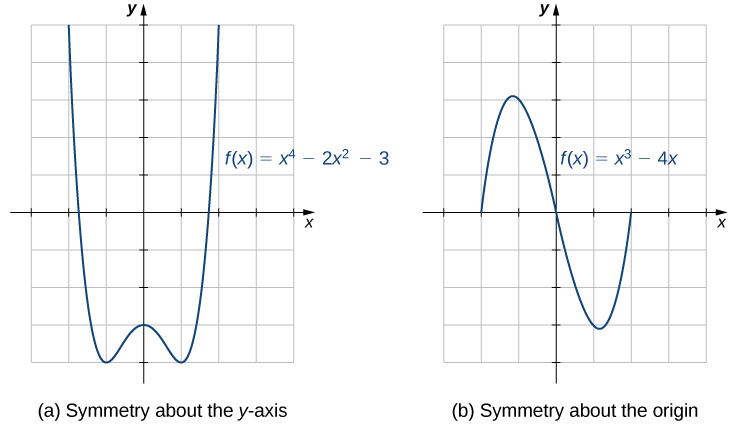Figure 12. (a) A graph that is symmetric about the $y$-axis. (b) A graph that is symmetric about the origin.

If we are given the graph of a function, it is easy to see whether the graph has one of these symmetry properties. But without a graph, how can we determine algebraically whether a function $f$ has symmetry? Looking at (Figure) again, we see that since $f$ is symmetric about the $y$-axis, if the point $(x,y)$ is on the graph, the point $(−x,y)$ is on the graph. In other words, $f(−x)=f(x)$. If a function $f$ has this property, we say $f$ is an even function, which has symmetry about the $y$-axis. For example, $f(x)=x^2$ is even because

$f(−x)=(−x)^2=x^2=f(x)$.

In contrast, looking at (Figure) again, if a function $f$ is symmetric about the origin, then whenever the point $(x,y)$ is on the graph, the point $(−x,−y)$ is also on the graph. In other words, $f(−x)=−f(x)$. If $f$ has this property, we say $f$ is an odd function, which has symmetry about the origin. For example, $f(x)=x^3$ is odd because

$f(−x)=(−x)^3=−x^3=−f(x)$.

### Definition

If $f(-x)=f(x)$ for all $x$ in the domain of $f$, then $f$ is an even function. An even function is symmetric about the $y$-axis.

If $f(−x)=−f(x)$ for all $x$ in the domain of $f$, then $f$ is an odd function. An odd function is symmetric about the origin.

### Even and Odd Functions

Determine whether each of the following functions is even, odd, or neither.

1. $f(x)=-5x^4+7x^2-2$
2. $f(x)=2x^5-4x+5$
3. $f(x)=\large{\frac{3x}{x^2+1}}$

Determine whether $f(x)=4x^3-5x$ is even, odd, or neither.

#### Hint

Compare $f(−x)$ with $f(x)$ and $−f(x)$.

One symmetric function that arises frequently is the absolute value function, written as $|x|$. The absolute value function is defined as

$f(x)=\begin{cases} x, & x \ge 0 \\ -x, & x < 0 \end{cases}$

Some students describe this function by stating that it “makes everything positive.” By the definition of the absolute value function, we see that if $x<0$, then $|x|=−x>0$, and if $x>0$, then $|x|=x>0$. However, for $x=0, \, |x|=0$. Therefore, it is more accurate to say that for all nonzero inputs, the output is positive, but if $x=0$, the output $|x|=0$. We conclude that the range of the absolute value function is $\{y|y\ge 0\}$. In (Figure), we see that the absolute value function is symmetric about the $y$-axis and is therefore an even function.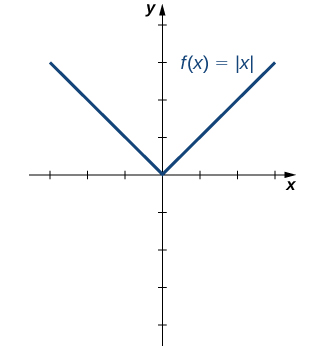Figure 13. The graph of $f(x)=|x|$ is symmetric about the $y$-axis.

### Working with the Absolute Value Function

Find the domain and range of the function $f(x)=2|x-3|+4$.

For the function $f(x)=|x+2|-4$, find the domain and range.

#### Hint

$|x+2|\ge 0$ for all real numbers $x$.

### Key Concepts

• A function is a mapping from a set of inputs to a set of outputs with exactly one output for each input.
• If no domain is stated for a function $y=f(x)$, the domain is considered to be the set of all real numbers $x$ for which the function is defined.
• When sketching the graph of a function $f$, each vertical line may intersect the graph, at most, once.
• A function may have any number of zeros, but it has, at most, one $y$-intercept.
• To define the composition $g\circ f$, the range of $f$ must be contained in the domain of $g$.
• Even functions are symmetric about the $y$-axis whereas odd functions are symmetric about the origin.

# Key Equations

• Composition of two functions
$(g\circ f)(x)=g(f(x))$
• Absolute value function
$f(x) = |x| = \begin{cases} x, & x \ge 0 \\ -x, & x < 0 \end{cases}$

For the following exercises, (a) determine the domain and the range of each relation, and (b) state whether the relation is a function.

1.

$x$ $y$ $x$ $y$
−3 9 1 1
−2 4 2 4
−1 1 3 9
0 0

2.

$x$ $y$ $x$ $y$
−3 −2 1 1
−2 −8 2 8
−1 −1 3 −2
0 0

3.

$x$ $y$ $x$ $y$
1 −3 1 1
2 −2 2 2
3 −1 3 3
0 0

4.

$x$ $y$ $x$ $y$
1 1 5 1
2 1 6 1
3 1 7 1
4 1

5.

$x$ $y$ $x$ $y$
3 3 15 1
5 2 21 2
8 1 33 3
10 0

6.

$x$ $y$ $x$ $y$
−7 11 1 −2
−2 5 3 4
−2 1 6 11
0 −1

For the following exercises, find the values for each function, if they exist, then simplify.

a. $f(0)$ b. $f(1)$ c. $f(3)$ d. $f(−x)$ e. $f(a)$ f. $f(a+h)$

7. $f(x)=5x-2$

8. $f(x)=4x^2-3x+1$

9. $f(x)=\frac{2}{x}$

10. $f(x)=|x-7|+8$

11. $f(x)=\sqrt{6x+5}$

12. $f(x)=\frac{x-2}{3x+7}$

13. $f(x)=9$

For the following exercises, find the domain, range, and all zeros/intercepts, if any, of the functions.

14. $f(x)=\frac{x}{x^2-16}$

15. $g(x)=\sqrt{8x-1}$

16. $h(x)=\frac{3}{x^2+4}$

17. $f(x)=-1+\sqrt{x+2}$

18. $f(x)=\frac{1}{\sqrt{x-9}}$

19. $g(x)=\frac{3}{x-4}$

20. $f(x)=4|x+5|$

21. $g(x)=\sqrt{\frac{7}{x-5}}$

For the following exercises, set up a table to sketch the graph of each function using the following values: $x=-3,-2,-1,0,1,2,3$.

22. $f(x)=x^2+1$

$x$ $y$ $x$ $y$
−3 10 1 2
−2 5 2 5
−1 2 3 10
0 1

23. $f(x)=3x-6$

$x$ $y$ $x$ $y$
−3 −15 1 −3
−2 −12 2 0
−1 −9 3 3
0 −6

24. $f(x)=\frac{1}{2}x+1$

$x$ $y$ $x$ $y$
−3 $-\frac{1}{2}$ 1 $\frac{3}{2}$
−2 0 2 2
−1 $\frac{1}{2}$ 3 $\frac{5}{2}$
0 1

25. $f(x)=2|x|$

$x$ $y$ $x$ $y$
−3 6 1 2
−2 4 2 4
−1 2 3 6
0 0

26. $f(x)=−x^2$

$x$ $y$ $x$ $y$
−3 −9 1 −1
−2 −4 2 −4
−1 −1 3 −9
0 0

27. $f(x)=x^3$

$x$ $y$ $x$ $y$
−3 −27 1 1
−2 −8 2 8
−1 −1 3 27
0 0

For the following exercises, use the vertical line test to determine whether each of the given graphs represents a function. Assume that a graph continues at both ends if it extends beyond the given grid. If the graph represents a function, then determine the following for each graph:

1. Domain and range
2. $x$-intercept, if any (estimate where necessary)
3. $y$-Intercept, if any (estimate where necessary)
4. The intervals for which the function is increasing
5. The intervals for which the function is decreasing
6. The intervals for which the function is constant
7. Symmetry about any axis and/or the origin
8. Whether the function is even, odd, or neither

28.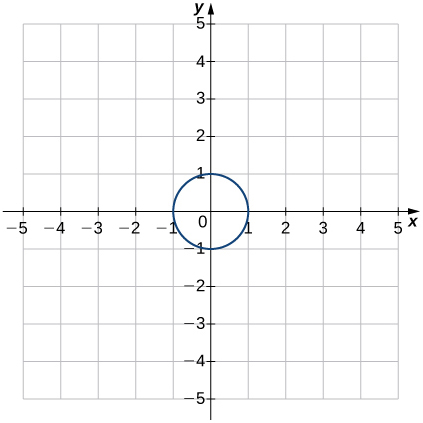29.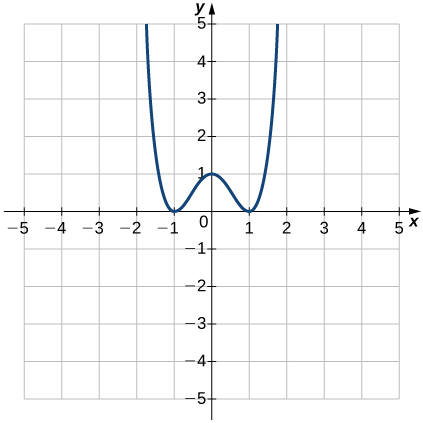30.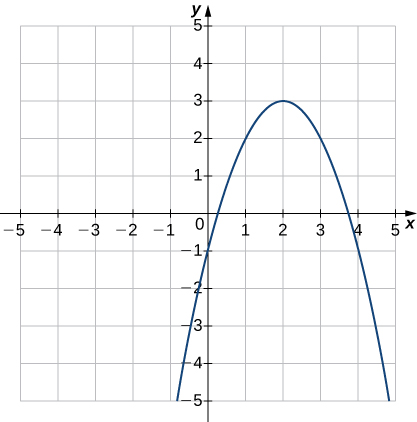31.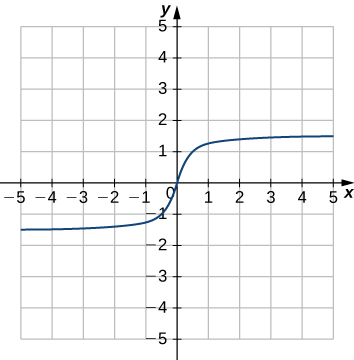32.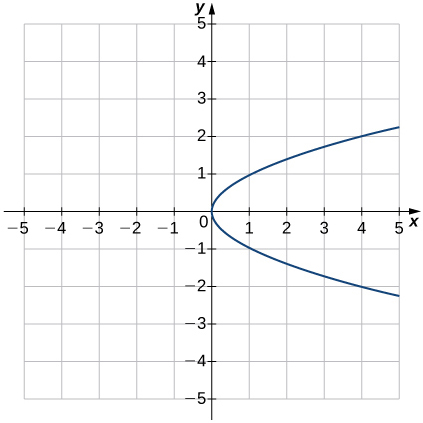33.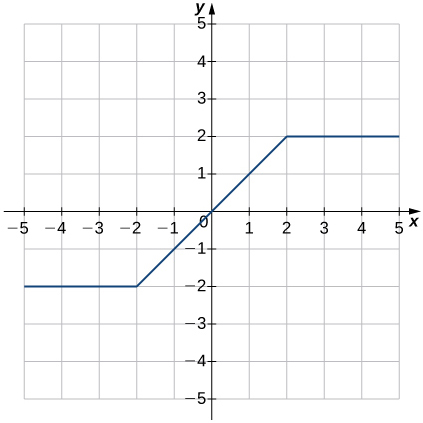34.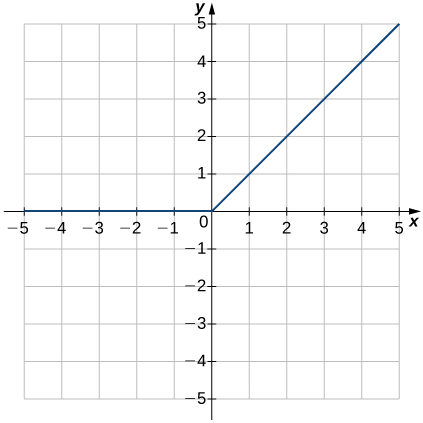35.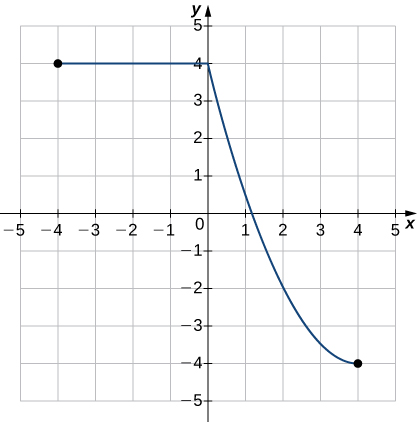For the following exercises, for each pair of functions, find a. $f+g$ b. $f-g$ c. $f·g$ d. $f/g$. Determine the domain of each of these new functions.

36. $f(x)=3x+4, \, g(x)=x-2$

37. $f(x)=x-8, \, g(x)=5x^2$

38. $f(x)=3x^2+4x+1, \, g(x)=x+1$

39. $f(x)=9-x^2, \, g(x)=x^2-2x-3$

40. $f(x)=\sqrt{x}, \, g(x)=x-2$

41. $f(x)=6+\frac{1}{x},g(x)=\frac{1}{x}$

For the following exercises, for each pair of functions, find a. $(f\circ g)(x)$ and b. $(g\circ f)(x)$ Simplify the results. Find the domain of each of the results.

42. $f(x)=3x, \, g(x)=x+5$

43. $f(x)=x+4, \, g(x)=4x-1$

44. $f(x)=2x+4, \, g(x)=x^2-2$

45. $f(x)=x^2+7, \, g(x)=x^2-3$

46. $f(x)=\sqrt{x}, \, g(x)=x+9$

47. $f(x)=\frac{3}{2x+1}, \, g(x)=\frac{2}{x}$

48. $f(x)=|x+1|, \, g(x)=x^2+x-4$

49. The table below lists the NBA championship winners for the years 2001 to 2012.

Year Winner
2001 LA Lakers
2002 LA Lakers
2003 San Antonio Spurs
2004 Detroit Pistons
2005 San Antonio Spurs
2006 Miami Heat
2007 San Antonio Spurs
2008 Boston Celtics
2009 LA Lakers
2010 LA Lakers
2011 Dallas Mavericks
2012 Miami Heat
1. Consider the relation in which the domain values are the years 2001 to 2012 and the range is the corresponding winner. Is this relation a function? Explain why or why not.
2. Consider the relation where the domain values are the winners and the range is the corresponding years. Is this relation a function? Explain why or why not.

50. [T] The area $A$ of a square depends on the length of the side $s$.

1. Write a function $A(s)$ for the area of a square.
2. Find and interpret $A(6.5)$.
3. Find the exact and the two-significant-digit approximation to the length of the sides of a square with area 56 square units.

51. [T] The volume of a cube depends on the length of the sides $s$.

1. Write a function $V(s)$ for the area of a square.
2. Find and interpret $V(11.8)$.

52. [T] A rental car company rents cars for a flat fee of $20 and an hourly charge of$10.25. Therefore, the total cost $C$ to rent a car is a function of the hours $t$ the car is rented plus the flat fee.

1. Write the formula for the function that models this situation.
2. Find the total cost to rent a car for 2 days and 7 hours.
3. Determine how long the car was rented if the bill is $432.73. 53. [T] A vehicle has a 20-gal tank and gets 15 mpg. The number of miles $N$ that can be driven depends on the amount of gas $x$ in the tank. 1. Write a formula that models this situation. 2. Determine the number of miles the vehicle can travel on (i) a full tank of gas and (ii) 3/4 of a tank of gas. 3. Determine the domain and range of the function. 4. Determine how many times the driver had to stop for gas if she has driven a total of 578 mi. 54. [T] The volume $V$ of a sphere depends on the length of its radius as $V=(4/3)\pi r^3$. Because Earth is not a perfect sphere, we can use the mean radius when measuring from the center to its surface. The mean radius is the average distance from the physical center to the surface, based on a large number of samples. Find the volume of Earth with mean radius $6.371×10^6$ m. 55. [T] A certain bacterium grows in culture in a circular region. The radius of the circle, measured in centimeters, is given by $r(t)=6-[5/(t^2+1)]$, where $t$ is time measured in hours since a circle of a 1 cm radius of the bacterium was put into the culture. 1. Express the area of the bacteria as a function of time. 2. Find the exact and approximate area of the bacterial culture in 3 hours. 3. Express the circumference of the bacteria as a function of time. 4. Find the exact and approximate circumference of the bacteria in 3 hours. 56. [T] An American tourist visits Paris and must convert U.S. dollars to Euros, which can be done using the function $E(x)=0.79x$, where $x$ is the number of U.S. dollars and $E(x)$ is the equivalent number of Euros. Since conversion rates fluctuate, when the tourist returns to the United States 2 weeks later, the conversion from Euros to U.S. dollars is $D(x)=1.245x$, where $x$ is the number of Euros and $D(x)$ is the equivalent number of U.S. dollars. 1. Find the composite function that converts directly from U.S. dollars to U.S. dollars via Euros. Did this tourist lose value in the conversion process? 2. Use (a) to determine how many U.S. dollars the tourist would get back at the end of her trip if she converted an extra$200 when she arrived in Paris.

57. [T] The manager at a skateboard shop pays his workers a monthly salary $S$ of $750 plus a commission of$8.50 for each skateboard they sell.

1. Write a function $y=S(x)$ that models a worker’s monthly salary based on the number of skateboards $x$ he or she sells.
2. Find the approximate monthly salary when a worker sells 25, 40, or 55 skateboards.
3. Use the INTERSECT feature on a graphing calculator to determine the number of skateboards that must be sold for a worker to earn a monthly income of \$1400. (Hint: Find the intersection of the function and the line $y=1400$.)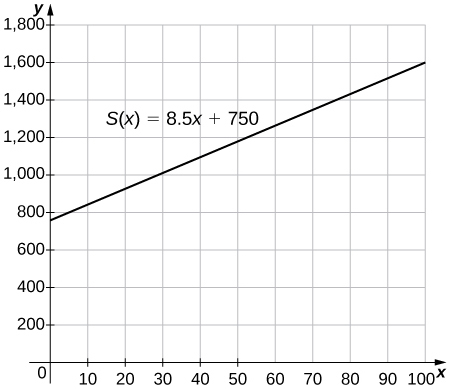58. [T] Use a graphing calculator to graph the half-circle $y=\sqrt{25-(x-4)^2}$. Then, use the INTERCEPT feature to find the value of both the $x$– and $y$-intercepts.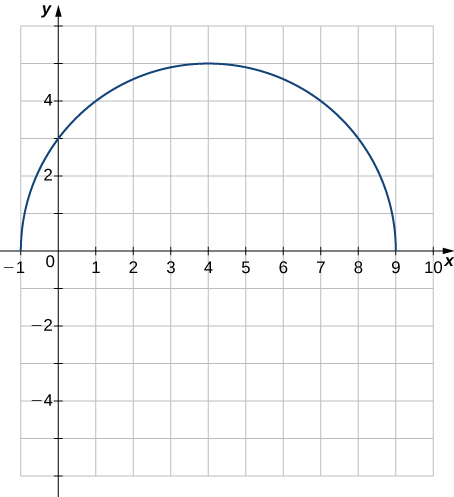## Glossary

absolute value function
$f(x) = |x| = \begin{cases} x, & x \ge 0 \\ -x, & x < 0 \end{cases}$
composite function
given two functions $f$ and $g$, a new function, denoted $g\circ f$, such that $(g\circ f)(x)=g(f(x))$
decreasing on the interval $I$
a function decreasing on the interval $I$ if, for all $x_1, \, x_2\in I, \, f(x_1)\ge f(x_2)$ if $x_1<x_2$
dependent variable
the output variable for a function
domain
the set of inputs for a function
even function
a function is even if $f(−x)=f(x)$ for all $x$ in the domain of $f$
function
a set of inputs, a set of outputs, and a rule for mapping each input to exactly one output
graph of a function
the set of points $(x,y)$ such that $x$ is in the domain of $f$ and $y=f(x)$
increasing on the interval $I$
a function increasing on the interval $I$ if for all $x_1, \, x_2\in I, \, f(x_1)\le f(x_2)$ if $x_1<x_2$
independent variable
the input variable for a function
odd function
a function is odd if $f(−x)=−f(x)$ for all $x$ in the domain of $f$
range
the set of outputs for a function
symmetry about the origin
the graph of a function $f$ is symmetric about the origin if $(−x,−y)$ is on the graph of $f$ whenever $(x,y)$ is on the graph
symmetry about the $y$-axis
the graph of a function $f$ is symmetric about the $y$-axis if $(−x,y)$ is on the graph of $f$ whenever $(x,y)$ is on the graph
table of values
a table containing a list of inputs and their corresponding outputs
vertical line test
given the graph of a function, every vertical line intersects the graph, at most, once
zeros of a function
when a real number $x$ is a zero of a function $f$, $f(x)=0$